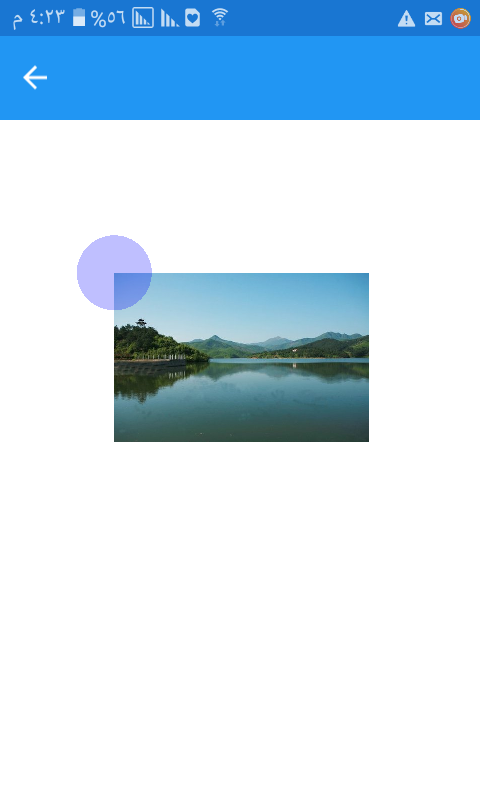# Use the scale with the touch point in skiasharp

Hi
This example is a modified version of Bezier Curve

```        SKBitmap bitmap;
public TestTouchPoint(SKBitmap bitmap)
{
this.bitmap = bitmap;
InitializeComponent();

touchPoints = new TouchPoint;
TouchPoint touchPoint1 = new TouchPoint();
touchPoint1.Center = new SKPoint(100, 100);
touchPoints = touchPoint1;
baseCanvasView = canvas;
}

private void Canvas_PaintSurface(object sender, SkiaSharp.Views.Forms.SKPaintSurfaceEventArgs e)
{
SKImageInfo info = e.Info;
SKSurface surface = e.Surface;
SKCanvas canvas = surface.Canvas;
canvas.Scale(0.5f);
canvas.Clear();

canvas.DrawBitmap(bitmap, touchPoints.Center.X, touchPoints.Center.Y);
foreach (TouchPoint touchPoint in touchPoints)
{
touchPoint.Paint(canvas);
}

}

```When i use

```canvas.Scale(0.5f);
```

The physical touchpoint of the screen will not match the touchpoint circle
How do I fix this so that the shapes match the position of the finger on the screen?

thanks

Tagged:

• I solved the problem by dividing the X and Y coordinates by the scale in each graphics functions

```  canvas.DrawBitmap(bitmap, touchPoints.Center.X / scale, touchPoints.Center.Y / scale);
```

. . .

```canvas.DrawCircle(touchPoints.Center.X / scale, touchPoints.Center.Y / scale, Radius / scale, touchPointPaint);
```

• use this:

```// Convert Xamarin.Forms point to pixels
Point pt = args.Location; //gets the point clicked
SKPoint point =
new SKPoint((float)(canvas.CanvasSize.Width * pt.X / canvas.Width),
(float)(canvas.CanvasSize.Height * pt.Y / canvas.Height));
var ratio = GetCurrentScale();
double double_X = point.X / ratio;
var XCoor = (decimal)double_X;

double double_Y = point.Y / ratio;
var YCoor = (decimal)double_Y;
```

Please note the above are useful for when you want to tailor for different scales/resolutions - you will need to install xamarin essentials nuget to get the current ratio.

This is the method for it:

private static double GetCurrentScale()
{
// Useful for rendering in the correct DPI
var displayInformation = DisplayInformation.GetForCurrentView();
var ratio = displayInformation.RawPixelsPerViewPixel;
return ratio;
}

Good luck

• I solved the problem by dividing the X and Y coordinates by the scale in each graphics functions

```  canvas.DrawBitmap(bitmap, touchPoints.Center.X / scale, touchPoints.Center.Y / scale);
```

. . .

```canvas.DrawCircle(touchPoints.Center.X / scale, touchPoints.Center.Y / scale, Radius / scale, touchPointPaint);
```